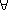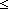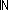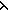IF YOU CAN SEE THIS go to /sfa/Nuprl/Shared/Xindentation_hack_doc.html

### Terms (Expressions)

Nuprl employs a generic form of expression, or term, for both primitive and defined operators. We often prefer the nomenclature "term" because "expression" connotes a meaningfulness that is not a priori assigned to these terms.

A term consists of: zero or more immediate subterms; for each subterm's place, a list of zero or more binding variables; and a nonempty list of non-term constituents, such as strings or numbers, which we call "operator parameters" or "op-parms" for reasons to be indicated below. For example, the term "x:A. 0x", has two immediate subterms, "A" and "0x", the variable "x" becoming bound in "0x" and no variables becoming bound in "A"; the only op-parm is the string "all", chosen for mnemonic purposes.

Normally one can infer the relevant term structure from the definitions provided for operators, or when the notation is standard or familiar. When we want to show the form of a term explicitly for some reason, we employ a uniform notation we have adopted for that purpose. For example, the form of "x:A. 0x" is

all{}(Ax.0x)

which shows that the first subterm is "A", with no variables becoming bound there, the second subterm is "0x", with the variable "x" becoming bound there, and there is just one op-parm "all". This first op-parm is called the "op-id".

The general form for this way of showing term structure is:

<opid>{<parms>}(<bterms>)

where <opid> is the first op-parm, usually thought of as (but not necessarily) identifying the operator; <parms> is a possibly empty sequence of op-parms; <bterms> is a sequence of "bound-terms" each consisting of a term and a list of binding variable names. When the <parms> or <bterms> is empty we often suppress the brackets surrounding them. Here we list several terms indicating both the structure revealing form, and the normal display.

 natnatural_number{4:n} 4 token{abc:t} "abc" variable{x:v} x variable{a:v}(x) a(x) (second-order variable instance) variable{a:v}(x; y) a(x;y) (second-order variable instance) add(a; b) a+b lambda(x.b)x.b all(A; x.B)x:A. B let(a; x.b) let x = a in b sym(A; x,y.B) Sym x,y:A. B decide(e; x.a; y.b) InjCase(e; x. a; y. b) wellfounded{i:l}(A; x,y.B) WellFnd{i}(A;x,y.B)

Any combination of term components forms a term. Note that there are several kinds of op-parm values, indicated after the colon in the above forms: [nat]:n [token]:t [string]:s [var]:v [level]:l.

See Computation.

(March 2001 - sfa )